## Two Sample t test for Comparing Two Means

Requirements: Two normally distributed but independent populations, σ is unknown

Hypothesis test

Formula:whereand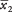are the means of the two samples, Δ is the hypothesized difference between the population means (0 if testing for equal means), s 1 and s 2are the standard deviations of the two samples, and n 1and n 2are the sizes of the two samples. The number of degrees of freedom for the problem is the smaller of n 1– 1 and n 2– 1.

An experiment is conducted to determine whether intensive tutoring (covering a great deal of material in a fixed amount of time) is more effective than paced tutoring (covering less material in the same amount of time). Two randomly chosen groups are tutored separately and then administered proficiency tests. Use a significance level of α < 0.05.

Let μ 1 represent the population mean for the intensive tutoring group and μ 2 represent the population mean for the paced tutoring group.

null hypothesis: H 0: μ 1 = μ 2

or H 0: μ 1 – μ 2 = 0

alternative hypothesis: H a : μ 1 > μ 2

or: H a : μ 1 – μ 2 > 0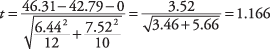The degrees of freedom parameter is the smaller of (12 – 1) and (10 – 1), or 9. Because this is a one‐tailed test, the alpha level (0.05) is not divided by two. The next step is to look up t .05,9in the t‐table (Table 3 in "Statistics Tables"), which gives a critical value of 1.833. The computed t of 1.166 does not exceed the tabled value, so the null hypothesis cannot be rejected. This test has not provided statistically significant evidence that intensive tutoring is superior to paced tutoring.

Formula:where a and b are the limits of the confidence interval,andare the means of the two samples,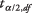is the value from the t‐table corresponding to half of the desired alpha level, s 1and s 2 are the standard deviations of the two samples, and n 1and n 2are the sizes of the two samples. The degrees of freedom parameter for looking up the t‐value is the smaller of n 1 – 1 and n 2– 1.

Estimate a 90 percent confidence interval for the difference between the number of raisins per box in two brands of breakfast cereal.The difference betweenandis 102.1 – 93.6 = 8.5. The degrees of freedom is the smaller of (6 – 1) and (9 – 1), or 5. A 90 percent confidence interval is equivalent to an alpha level of 0.10, which is then halved to give 0.05. According to Table 3 in "Statistics Tables," the critical value for t .05,5 is 2.015. The interval may now be computed.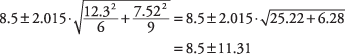The interval is (–2.81, 19.81).

You can be 90 percent confident that Brand A cereal has between 2.81 fewer and 19.81 more raisins per box than Brand B. The fact that the interval contains 0 means that if you had performed a test of the hypothesis that the two population means are different (using the same significance level), you would not have been able to reject the null hypothesis of no difference.

If the two population distributions can be assumed to have the same variance—and, therefore, the same standard deviation— s 1and s 2 can be pooled together, each weighted by the number of cases in each sample. Although using pooled variance in a t‐test is generally more likely to yield significant results than using separate variances, it is often hard to know whether the variances of the two populations are equal. For this reason, the pooled variance method should be used with caution. The formula for the pooled estimator of σ 2 is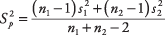where s 1and s 2are the standard deviations of the two samples and n 1 and n 2are the sizes of the two samples.

The formula for comparing the means of two populations using pooled variance is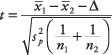where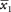andare the means of the two samples, Δ is the hypothesized difference between the population means (0 if testing for equal means), s p 2 is the pooled variance, and n 1and n 2are the sizes of the two samples. The number of degrees of freedom for the problem is

df = n 1+ n 2– 2

Does right‐ or left‐handedness affect how fast people type? Random samples of students from a typing class are given a typing speed test (words per minute), and the results are compared. Significance level for the test: 0.10. Because you are looking for a difference between the groups in either direction (right‐handed faster than left, or vice versa), this is a two‐tailed test.

null hypothesis: H 0: μ 1 = μ 2

or: H 0: μ 1 – μ 2 = 0

alternative hypothesis: H a : μ 1 ≠ μ 2

or: H a : μ 1 – μ 2 ≠ 0First, calculate the pooled variance: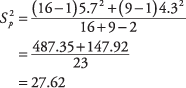Next, calculate the t‐value:The degrees‐of freedom parameter is 16 + 9 – 2, or 23. This test is a two‐tailed one, so you divide the alpha level (0.10) by two. Next, you look up t .05,23in the t‐table (Table 3 in "Statistics Tables"), which gives a critical value

of 1.714. This value is larger than the absolute value of the computed t of –1.598, so the null hypothesis of equal population means cannot be rejected. There is no evidence that right‐ or left handedness has any effect on typing speed.